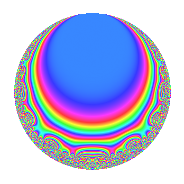# Properties

 Label 24.4.dLevel 24 Weight 4 Character orbit d Rep. character $$\chi_{24}(13,\cdot)$$ Character field $$\Q$$ Dimension 6 Newforms 1 Sturm bound 16 Trace bound 0

# Related objects

## Defining parameters

 Level: $$N$$ = $$24 = 2^{3} \cdot 3$$ Weight: $$k$$ = $$4$$ Character orbit: $$[\chi]$$ = 24.d (of order $$2$$ and degree $$1$$) Character conductor: $$\operatorname{cond}(\chi)$$ = $$8$$ Character field: $$\Q$$ Newforms: $$1$$ Sturm bound: $$16$$ Trace bound: $$0$$

## Dimensions

The following table gives the dimensions of various subspaces of $$M_{4}(24, [\chi])$$.

Total New Old
Modular forms 14 6 8
Cusp forms 10 6 4
Eisenstein series 4 0 4

## Trace form

 $$6q$$ $$\mathstrut +\mathstrut 2q^{2}$$ $$\mathstrut +\mathstrut 16q^{4}$$ $$\mathstrut -\mathstrut 6q^{6}$$ $$\mathstrut +\mathstrut 28q^{7}$$ $$\mathstrut -\mathstrut 76q^{8}$$ $$\mathstrut -\mathstrut 54q^{9}$$ $$\mathstrut +\mathstrut O(q^{10})$$ $$6q$$ $$\mathstrut +\mathstrut 2q^{2}$$ $$\mathstrut +\mathstrut 16q^{4}$$ $$\mathstrut -\mathstrut 6q^{6}$$ $$\mathstrut +\mathstrut 28q^{7}$$ $$\mathstrut -\mathstrut 76q^{8}$$ $$\mathstrut -\mathstrut 54q^{9}$$ $$\mathstrut +\mathstrut 60q^{10}$$ $$\mathstrut -\mathstrut 12q^{12}$$ $$\mathstrut -\mathstrut 100q^{14}$$ $$\mathstrut -\mathstrut 60q^{15}$$ $$\mathstrut +\mathstrut 56q^{16}$$ $$\mathstrut +\mathstrut 52q^{17}$$ $$\mathstrut -\mathstrut 18q^{18}$$ $$\mathstrut +\mathstrut 56q^{20}$$ $$\mathstrut +\mathstrut 224q^{22}$$ $$\mathstrut +\mathstrut 328q^{23}$$ $$\mathstrut +\mathstrut 204q^{24}$$ $$\mathstrut -\mathstrut 106q^{25}$$ $$\mathstrut +\mathstrut 56q^{26}$$ $$\mathstrut -\mathstrut 352q^{28}$$ $$\mathstrut +\mathstrut 372q^{30}$$ $$\mathstrut -\mathstrut 636q^{31}$$ $$\mathstrut -\mathstrut 248q^{32}$$ $$\mathstrut -\mathstrut 548q^{34}$$ $$\mathstrut -\mathstrut 144q^{36}$$ $$\mathstrut -\mathstrut 776q^{38}$$ $$\mathstrut +\mathstrut 312q^{39}$$ $$\mathstrut +\mathstrut 232q^{40}$$ $$\mathstrut +\mathstrut 236q^{41}$$ $$\mathstrut -\mathstrut 564q^{42}$$ $$\mathstrut +\mathstrut 1152q^{44}$$ $$\mathstrut +\mathstrut 328q^{46}$$ $$\mathstrut -\mathstrut 408q^{47}$$ $$\mathstrut +\mathstrut 576q^{48}$$ $$\mathstrut +\mathstrut 654q^{49}$$ $$\mathstrut +\mathstrut 1970q^{50}$$ $$\mathstrut -\mathstrut 368q^{52}$$ $$\mathstrut +\mathstrut 54q^{54}$$ $$\mathstrut +\mathstrut 1024q^{55}$$ $$\mathstrut -\mathstrut 1864q^{56}$$ $$\mathstrut -\mathstrut 168q^{57}$$ $$\mathstrut +\mathstrut 140q^{58}$$ $$\mathstrut -\mathstrut 1152q^{60}$$ $$\mathstrut -\mathstrut 2108q^{62}$$ $$\mathstrut -\mathstrut 252q^{63}$$ $$\mathstrut +\mathstrut 832q^{64}$$ $$\mathstrut -\mathstrut 1744q^{65}$$ $$\mathstrut -\mathstrut 1440q^{66}$$ $$\mathstrut +\mathstrut 2976q^{68}$$ $$\mathstrut +\mathstrut 1352q^{70}$$ $$\mathstrut -\mathstrut 1704q^{71}$$ $$\mathstrut +\mathstrut 684q^{72}$$ $$\mathstrut +\mathstrut 956q^{73}$$ $$\mathstrut +\mathstrut 1568q^{74}$$ $$\mathstrut -\mathstrut 1744q^{76}$$ $$\mathstrut +\mathstrut 1608q^{78}$$ $$\mathstrut -\mathstrut 44q^{79}$$ $$\mathstrut -\mathstrut 2112q^{80}$$ $$\mathstrut +\mathstrut 486q^{81}$$ $$\mathstrut -\mathstrut 2236q^{82}$$ $$\mathstrut -\mathstrut 1992q^{84}$$ $$\mathstrut -\mathstrut 760q^{86}$$ $$\mathstrut +\mathstrut 1044q^{87}$$ $$\mathstrut +\mathstrut 1856q^{88}$$ $$\mathstrut -\mathstrut 220q^{89}$$ $$\mathstrut -\mathstrut 540q^{90}$$ $$\mathstrut +\mathstrut 1728q^{92}$$ $$\mathstrut +\mathstrut 2088q^{94}$$ $$\mathstrut +\mathstrut 5104q^{95}$$ $$\mathstrut +\mathstrut 2184q^{96}$$ $$\mathstrut -\mathstrut 2444q^{97}$$ $$\mathstrut +\mathstrut 3354q^{98}$$ $$\mathstrut +\mathstrut O(q^{100})$$

## Decomposition of $$S_{4}^{\mathrm{new}}(24, [\chi])$$ into irreducible Hecke orbits

Label Dim. $$A$$ Field CM Traces $q$-expansion
$$a_2$$ $$a_3$$ $$a_5$$ $$a_7$$
24.4.d.a $$6$$ $$1.416$$ 6.0.8248384.1 None $$2$$ $$0$$ $$0$$ $$28$$ $$q+\beta _{3}q^{2}+\beta _{1}q^{3}+(3-\beta _{5})q^{4}+(2\beta _{1}+\cdots)q^{5}+\cdots$$

## Decomposition of $$S_{4}^{\mathrm{old}}(24, [\chi])$$ into lower level spaces

$$S_{4}^{\mathrm{old}}(24, [\chi]) \cong$$ $$S_{4}^{\mathrm{new}}(8, [\chi])$$$$^{\oplus 2}$$